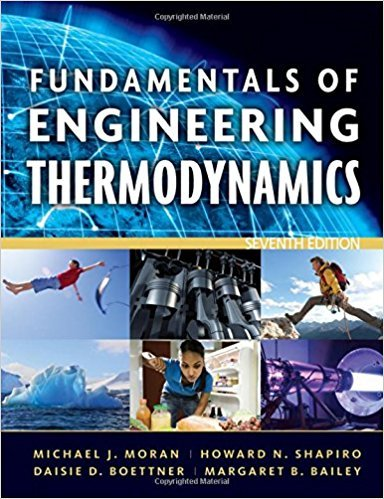×
Get Full Access to Fundamentals Of Engineering Thermodynamics - 7 Edition - Chapter 2 - Problem 91p
Get Full Access to Fundamentals Of Engineering Thermodynamics - 7 Edition - Chapter 2 - Problem 91p

×

# Analyzing Thermodynamic CyclesA heat pump maintains aISBN: 9780470495902 50

## Solution for problem 91P Chapter 2

Fundamentals of Engineering Thermodynamics | 7th Edition

• Textbook Solutions
• 2901 Step-by-step solutions solved by professors and subject experts
• Get 24/7 help from StudySoup virtual teaching assistantsFundamentals of Engineering Thermodynamics | 7th Edition

4 5 1 402 Reviews
16
5
Problem 91P

Problem 91P

Analyzing Thermodynamic Cycles

A heat pump maintains a dwelling at 68°F. When operating steadily, the power input to the heat pump is 5 hp, and the heat pump receives energy by heat transfer from 55°F well water at a rate of 500 Btu/min.

(a) Determine the coefficient of performance.

(b) Evaluating electricity at \$ 0.10 per kW · h, determine the cost of electricity in a month when the heat pump operates for 300 hours.

Step-by-Step Solution:

a.)

Step 1 of 2

We have to determine the coefficient of performance for the heat pump.

The coefficient of performance is given by the expressionWhere,= is the energy discharged by heat transfer from the system
to the hot body= heat energy the pump receives by heat transfer from the well

= 500 Btu/min= net work input  = 5 hpSo,Now,Hence, the coefficient of performance isThus, the coefficient of performance is 3.3.

b.)

Step 2 of 2

##### ISBN: 9780470495902

This textbook survival guide was created for the textbook: Fundamentals of Engineering Thermodynamics, edition: 7. Fundamentals of Engineering Thermodynamics was written by and is associated to the ISBN: 9780470495902. The full step-by-step solution to problem: 91P from chapter: 2 was answered by , our top Engineering and Tech solution expert on 07/20/17, 09:01AM. This full solution covers the following key subjects: heat, Pump, determine, electricity, min. This expansive textbook survival guide covers 14 chapters, and 1501 solutions. The answer to “Analyzing Thermodynamic CyclesA heat pump maintains a dwelling at 68°F. When operating steadily, the power input to the heat pump is 5 hp, and the heat pump receives energy by heat transfer from 55°F well water at a rate of 500 Btu/min.(a) Determine the coefficient of performance.________________(b) Evaluating electricity at \$ 0.10 per kW · h, determine the cost of electricity in a month when the heat pump operates for 300 hours.” is broken down into a number of easy to follow steps, and 72 words. Since the solution to 91P from 2 chapter was answered, more than 782 students have viewed the full step-by-step answer.

Unlock Textbook Solution